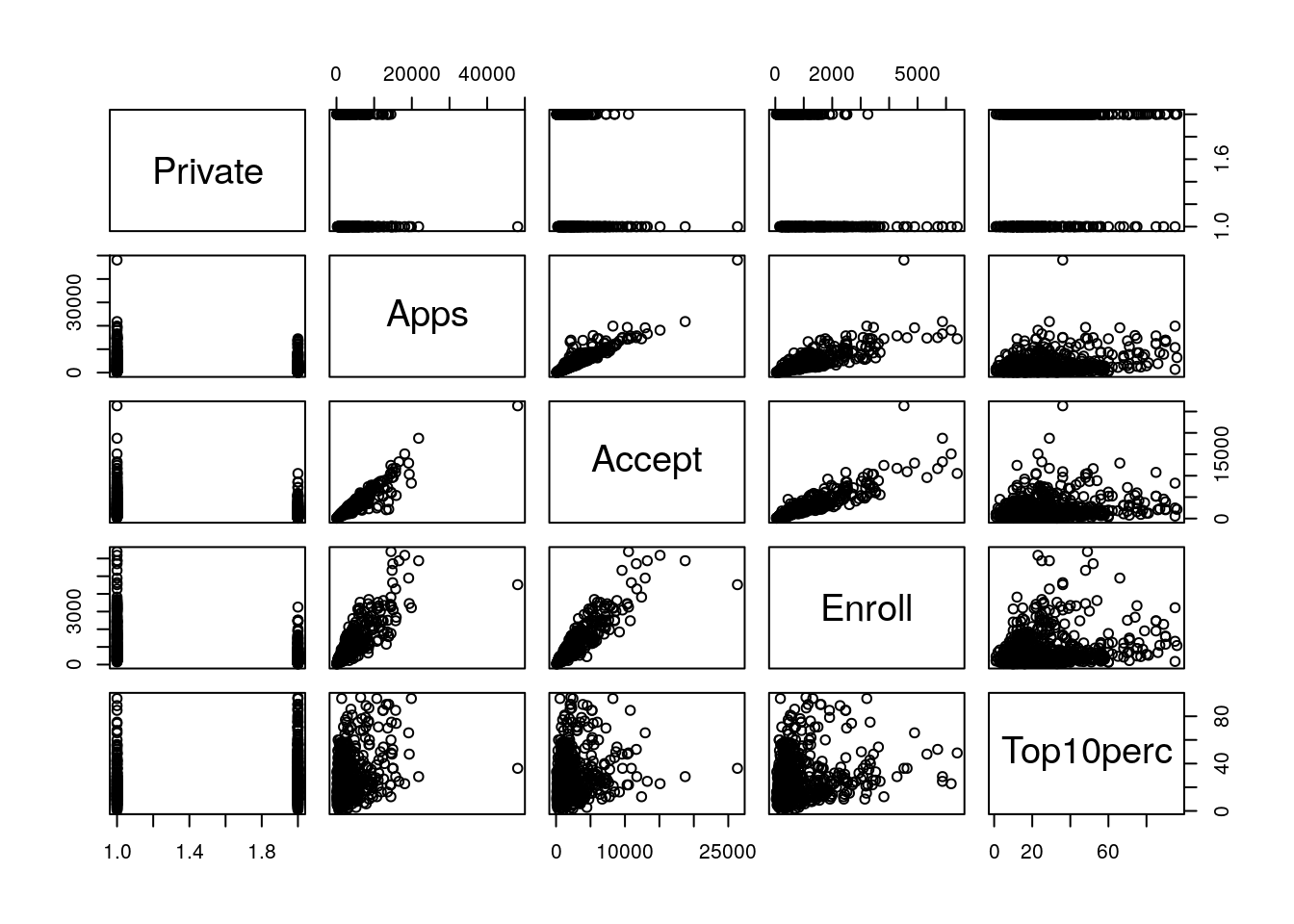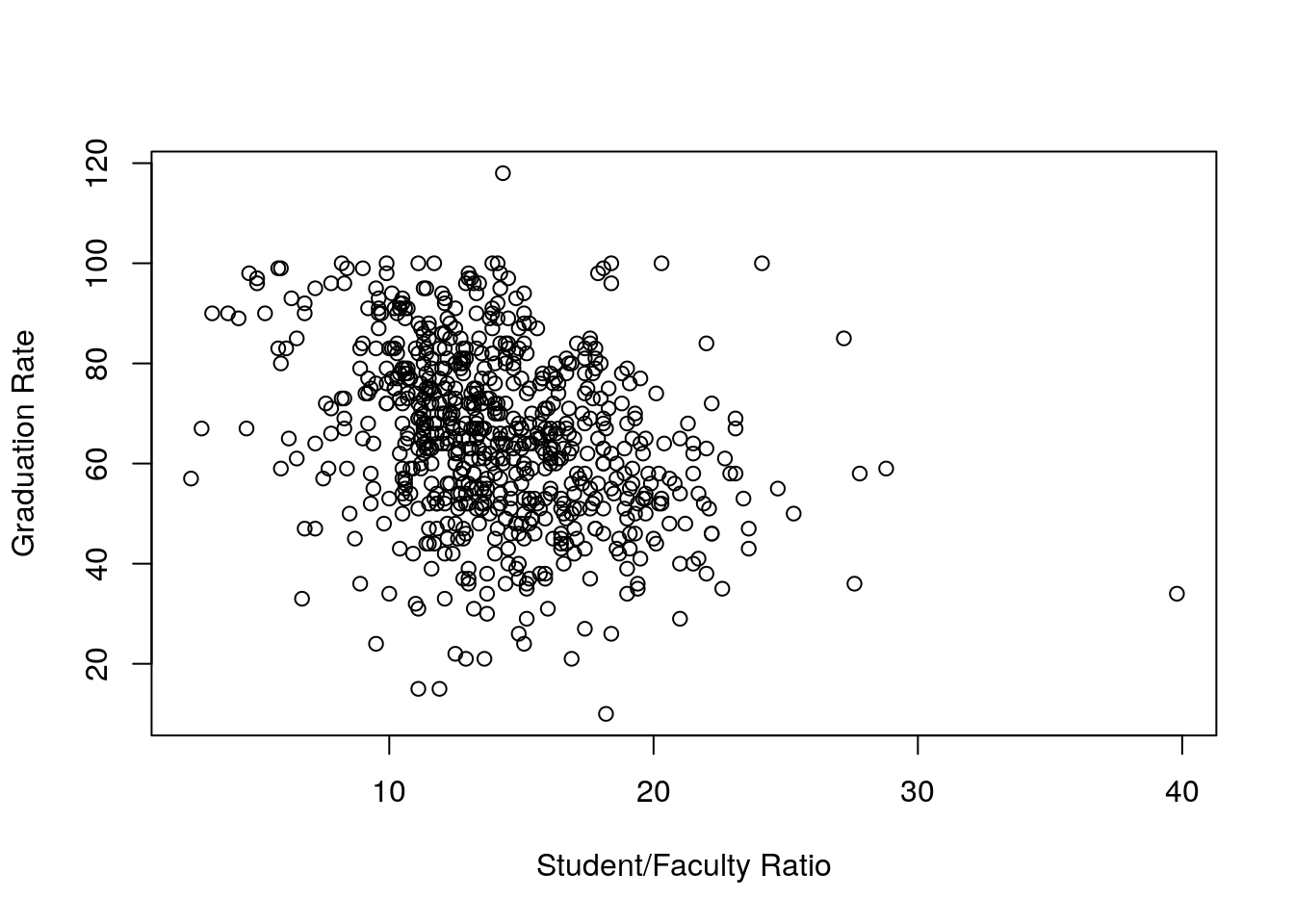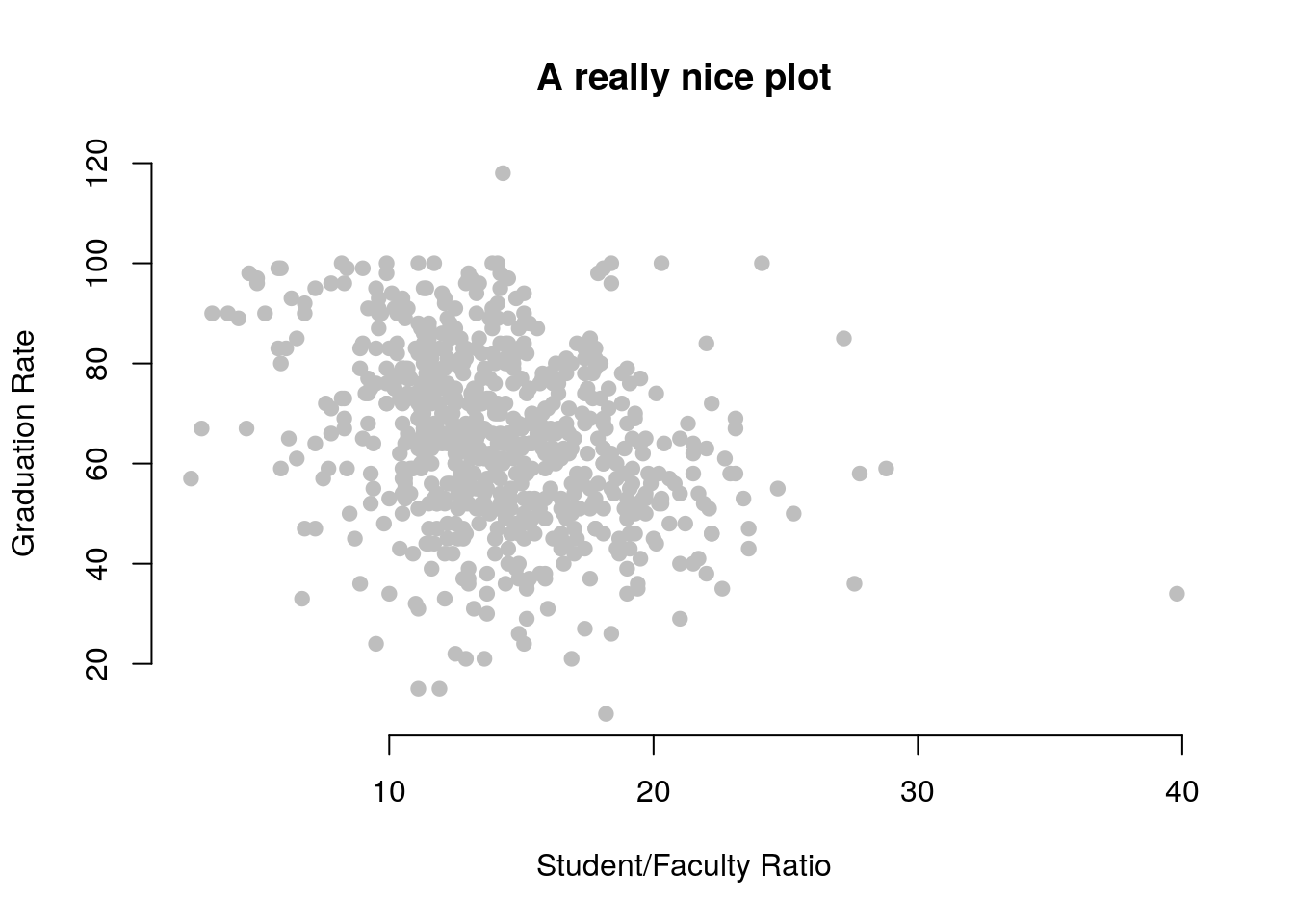### Exercise 1

Open RStudio and explore the programme. Make sure you can identify the `console`, the script editor, the `environment` window, the `packages` window and the `help` window!

1. In the script window, write code that creates a vector composed of 5 single-digit integers. To concatinate a series of values, use the `c()` function, with each argument separated by a comma. So, a character vector of length three could be `c("a", "b", "c")`. Assign your integer vector using the assignment operator `<-` to an object with a name of your choosing.
``my_vec <- c(1,2,3,4,5)``
1. Multiply your vector by 3, and assign the output to a new object. Print the values of your new object.
``````my_new_vec <- my_vec * 3
print(my_new_vec)``````
``##   3  6  9 12 15``
1. Add together the two objects that you have created to far, printing the result. Note that R operates on vectors element-wise.
``print(my_new_vec + my_vec)``
``##   4  8 12 16 20``
1. Create a logical vector of length five, again using the `c()` function. Make sure that you have a mix of `TRUE` and `FALSE` values in the vector. Use the logical vector to subset the numeric vector that you created in question 2 and `print` the result.
``````my_logical_vec <- c(T, T, T, F, F)
print(my_new_vec[my_logical_vec])``````
``##  3 6 9``
1. Subset to just the first two elements of the numeric vector that you created in question 2 and assign the result to have the name `my_short_vector`.
``my_short_vector <- my_new_vec[c(1,2)]``

### Exercise 2

This exercise relates to the `College` data set, which comes from An Introduction to Statistical Learning by James et al 2013.. It contains a number of variables for 777 different universities and colleges in the US.

The variables are

• `Private` : Public/private indicator
• `Apps` : Number of applications received
• `Accept` : Number of applicants accepted
• `Enroll` : Number of new students enrolled
• `Top10perc` : New students from top 10% of high school class
• `Top25perc` : New students from top 25% of high school class
• `F.Undergrad` : Number of full-time undergraduates
• `P.Undergrad` : Number of part-time undergraduates
• `Outstate` : Out-of-state tuition
• `Room.Board` : Room and board costs
• `Books` : Estimated book costs
• `Personal` : Estimated personal spending
• `PhD` : Percent of faculty with Ph.D.’s
• `Terminal` : Percent of faculty with terminal degree
• `S.F.Ratio` : Student/faculty ratio
• `perc.alumni` : Percent of alumni who donate
• `Expend` : Instructional expenditure per student
• `Grad.Rate` : Graduation rate

You can either download the .csv file containing the data from the MY591 moodle page, or read the data in directly from the website.

1. Use the `read.csv()` function to read the data into `R`. Call the loaded data `college`. Make sure that you have the directory set to the correct location for the data. You can load this in R directly from the website, using:
``college <- read.csv("http://www-bcf.usc.edu/~gareth/ISL/College.csv")``

Or you can load it from a saved file, using:

``college <- read.csv("path_to_my_file/College.csv")``
1. Look at the data using the `View()` function. You should notice that the first column is just the name of each university. We don’t really want `R` to treat this as data. However, it may be handy to have these names for later. Try the following commands:
``````rownames(college) <- college[, 1]
View(college)``````

You should see that there is now a `row.names` column with the name of each university recorded. This means that `R` has given each row a name corresponding to the appropriate university. `R` will not try to perform calculations on the row names. However, we still need to eliminate the first column in the data where the names are stored. Try

``````college <- college[, -1]
View(college)``````

Now you should see that the first data column is `Private`. Note that another column labeled `row.names` now appears before the `Private` column. However, this is not a data column but rather the name that `R` is giving to each row.

1. Use the `str()` function to look at the structure of the data. Which of the variables are numeric? Which are integer? Which are factors?
``str(college)``
``````## 'data.frame':    777 obs. of  18 variables:
##  \$ Private    : Factor w/ 2 levels "No","Yes": 2 2 2 2 2 2 2 2 2 2 ...
##  \$ Apps       : int  1660 2186 1428 417 193 587 353 1899 1038 582 ...
##  \$ Accept     : int  1232 1924 1097 349 146 479 340 1720 839 498 ...
##  \$ Enroll     : int  721 512 336 137 55 158 103 489 227 172 ...
##  \$ Top10perc  : int  23 16 22 60 16 38 17 37 30 21 ...
##  \$ Top25perc  : int  52 29 50 89 44 62 45 68 63 44 ...
##  \$ F.Undergrad: int  2885 2683 1036 510 249 678 416 1594 973 799 ...
##  \$ P.Undergrad: int  537 1227 99 63 869 41 230 32 306 78 ...
##  \$ Outstate   : int  7440 12280 11250 12960 7560 13500 13290 13868 15595 10468 ...
##  \$ Room.Board : int  3300 6450 3750 5450 4120 3335 5720 4826 4400 3380 ...
##  \$ Books      : int  450 750 400 450 800 500 500 450 300 660 ...
##  \$ Personal   : int  2200 1500 1165 875 1500 675 1500 850 500 1800 ...
##  \$ PhD        : int  70 29 53 92 76 67 90 89 79 40 ...
##  \$ Terminal   : int  78 30 66 97 72 73 93 100 84 41 ...
##  \$ S.F.Ratio  : num  18.1 12.2 12.9 7.7 11.9 9.4 11.5 13.7 11.3 11.5 ...
##  \$ perc.alumni: int  12 16 30 37 2 11 26 37 23 15 ...
##  \$ Expend     : int  7041 10527 8735 19016 10922 9727 8861 11487 11644 8991 ...
##  \$ Grad.Rate  : int  60 56 54 59 15 55 63 73 80 52 ...``````
1. Use the `summary()` function to produce a numerical summary of the variables in the data set.
``summary(college)``
``````##  Private        Apps           Accept          Enroll       Top10perc
##  No :212   Min.   :   81   Min.   :   72   Min.   :  35   Min.   : 1.00
##  Yes:565   1st Qu.:  776   1st Qu.:  604   1st Qu.: 242   1st Qu.:15.00
##            Median : 1558   Median : 1110   Median : 434   Median :23.00
##            Mean   : 3002   Mean   : 2019   Mean   : 780   Mean   :27.56
##            3rd Qu.: 3624   3rd Qu.: 2424   3rd Qu.: 902   3rd Qu.:35.00
##            Max.   :48094   Max.   :26330   Max.   :6392   Max.   :96.00
##  Min.   :  9.0   Min.   :  139   Min.   :    1.0   Min.   : 2340
##  1st Qu.: 41.0   1st Qu.:  992   1st Qu.:   95.0   1st Qu.: 7320
##  Median : 54.0   Median : 1707   Median :  353.0   Median : 9990
##  Mean   : 55.8   Mean   : 3700   Mean   :  855.3   Mean   :10441
##  3rd Qu.: 69.0   3rd Qu.: 4005   3rd Qu.:  967.0   3rd Qu.:12925
##  Max.   :100.0   Max.   :31643   Max.   :21836.0   Max.   :21700
##    Room.Board       Books           Personal         PhD
##  Min.   :1780   Min.   :  96.0   Min.   : 250   Min.   :  8.00
##  1st Qu.:3597   1st Qu.: 470.0   1st Qu.: 850   1st Qu.: 62.00
##  Median :4200   Median : 500.0   Median :1200   Median : 75.00
##  Mean   :4358   Mean   : 549.4   Mean   :1341   Mean   : 72.66
##  3rd Qu.:5050   3rd Qu.: 600.0   3rd Qu.:1700   3rd Qu.: 85.00
##  Max.   :8124   Max.   :2340.0   Max.   :6800   Max.   :103.00
##     Terminal       S.F.Ratio      perc.alumni        Expend
##  Min.   : 24.0   Min.   : 2.50   Min.   : 0.00   Min.   : 3186
##  1st Qu.: 71.0   1st Qu.:11.50   1st Qu.:13.00   1st Qu.: 6751
##  Median : 82.0   Median :13.60   Median :21.00   Median : 8377
##  Mean   : 79.7   Mean   :14.09   Mean   :22.74   Mean   : 9660
##  3rd Qu.: 92.0   3rd Qu.:16.50   3rd Qu.:31.00   3rd Qu.:10830
##  Max.   :100.0   Max.   :39.80   Max.   :64.00   Max.   :56233
##  Min.   : 10.00
##  1st Qu.: 53.00
##  Median : 65.00
##  Mean   : 65.46
##  3rd Qu.: 78.00
##  Max.   :118.00``````
1. What is the mean and standard deviation of the `Enroll` and `Top10Perc` variables?
``mean(college\$Enroll)``
``##  779.973``
``mean(college\$Top10perc)``
``##  27.55856``
``sd(college\$Enroll)``
``##  929.1762``
``sd(college\$Top10perc)``
``##  17.64036``
1. Now remove the 10th through 85th observations. What is the mean and standard deviation of the `Enroll` and `Top10Perc` variables in the subset of the data that remains?
``````college <- college[-c(15:85),]
mean(college\$Enroll)``````
``##  784.8867``
``mean(college\$Top10perc)``
``##  27.51841``
``sd(college\$Enroll)``
``##  928.6599``
``sd(college\$Top10perc)``
``##  17.61432``
1. What is the range of the `Books` variable?
``range(college\$Books)``
``##   110 2340``
1. Use the `pairs()` function to produce a scatterplot matrix of the first five columns or variables of the data. Recall that you can reference the first five columns of a matrix `A` using `A[,1:5]`.
``pairs(college[,1:5])``1. Use the `plot()` function to produce a scatter plot of `S.F.Ratio` versus `Grad.Rate`. Give the axes informative labels.
``plot(college\$S.F.Ratio, college\$Grad.Rate, xlab = "Student/Faculty Ratio", ylab = "Graduation Rate")``1. Compete with your neighbour to make the prettiest plot. You might want to look at `?plot` and `?par` for some ideas. If you are feeling very keen, try using `ggplot` but you will need to load the ggplot library first: `library(ggplot2)`.
``````plot(college\$S.F.Ratio, college\$Grad.Rate,
xlab = "Student/Faculty Ratio", ylab = "Graduation Rate",
main = "A really nice plot",
pch = 19, col = "gray", bty = "n")````````````library(ggplot2)
ggplot(data = college, aes(x = S.F.Ratio, y = Grad.Rate, col = Private)) +
geom_point()+
xlab("Student/Faculty Ratio")+